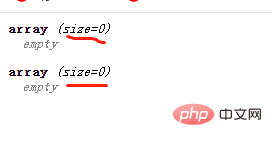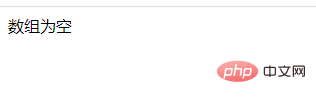# php中什么叫空数组php中的空数组

```<?php
\$arr1=array();
\$arr2=[];
var_dump(\$arr1);
var_dump(\$arr2);
?>```1、使用count()函数判断是否为空数组。

`count(\$arr,\$m)`函数是用于计算数组中的所有元素。如果数组中的元素数为零，则它将显示空数组。

\$m：为可选参数，可以省略。

• 如果省略 \$m 参数，或者将其设置为 COUNT_NORMAL 或者 0，count() 函数将不检测多维数组；

• 如果 \$m 设置为 COUNT_RECURSIVE 或者 1，count() 函数将递归计算数组中元素的个数，对于计算多维数组的元素个数尤为有用。

```<?php
// 声明一个空数组
\$empty_array = array();

// 检查数组是否为空
if(count(\$empty_array) == 0)
echo "数组为空";
else
echo "数组不为空";
?>```2、使用sizeof()函数判断是否为空数组。

`sizeof(\$arr,\$m)` 函数是 count() 函数的别名，也就是所 sizeof() 函数的功能及使用方法与 count() 函数完全相同。

```<?php
// 声明一个空数组
\$empty_array = array();

if( sizeof(\$empty_array) == 0 )
echo "数组为空";
else
echo "数组不空";
?>```

`数组为空`• 相关标签：php数组 php
• 相关文章

相关视频